# Calculating the Equilibrium Constants

Calculating Kc from Known Equilibrium Amounts

• Write the equilibrium expression for the reaction.
• Determine the molar concentrations or partial pressures of each species involved.
• Subsititute into the equilibrium expression and solve for Kc.

Example: Calculate the value of the equilibrium constant, Kc , for the system shown, if 0.1908 moles of CO2, 0.0908 moles of H2, 0.0092 moles of CO, and 0.0092 moles of H2O vapor were present in a 2.00 L reaction vessel were present at equilibrium.• Since Kc is being determined, check to see if the given equilibrium amounts are expressed in moles per liter (molarity).  In this example they are not; conversion of each is requried.[CO2] = 0.1908 mol CO2/2.00 L = 0.0954 M
[H2] = 0.0454 M
[CO] = 0.0046 M
[H2O] = 0.0046 M
• Substitute each concentration into the equilibrium expression and calculate the value of the equilibrium constant.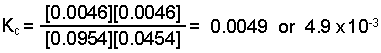Top

Calculating K from Initial Amounts and One Known Equilibrium Amount

• Write the equilibrium expression for the reaction.
• Determine the molar concentrations or partial pressures of each species involved.
• Determine all equilibrium concentrations or partial pressures using an ICE chart.
• Substitute into the equilibrium expression and solve for Kc

Example:   Initially, a mixture of 0.100 M NO, 0.050 M H2, 0.100 M H2 O was allowed to reach equilibrium (initially there was no N2 ).  At equilibrium the concentration of NO was found to be 0.062 M.  Determine the value of the equilibrium constant, Kc , for the reaction: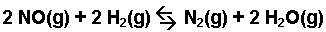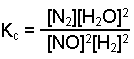• Check to see if the amounts are expressed in moles per liter (molarity) since Kc is being .  In this example they are.
• Create an ICE chart that expresses the initial concentration, the change in concentration, and the equilibrium concentration for each species in the reaction.  From the chart you can determine the changes in the concentrations of each species and the equilibrium concentrations.  From the example, we start with the folowing information.
 NO H2 N2 H2O Initial Concentration (M) 0.100 0.0500 0 0.100 Change in Concentration (M) – 2 x – 2 x + x + 2 x Equilibrium Concentration (M) 0.062

The change in concentration of the NO was (0.062 M – 0.100M) = – 0.038 M.  Thus -2 x = – 0.038 and x = 0.019. Note: the negative sign indicates a decreasing concentration, not a negative concentration.  The changes in the other species must agree with the stoichiometry dictated by the balance equation.  The hydrogen will also change by – 0.038 M, while the nitrogen will increase by + 0.019 M and the water will increase by + 0.038 M.  From these changes we can complete the chart to find the equilibrium concentrations for each species.

 NO H2 N2 H2O Initial Concentration (M) 0.100 0.0500 0 0.100 Change in Concentration (M) – 0.038 – 0.038 + 0.019 + 0.038 Equilibrium Concentration (M) 0.062 0.012 0.019 0.138
• Substitute the equilibrium concentrations into the equilibrium expression and solve for Kc.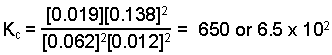Top

Calculating Kc from Known Initial Amounts and the Known Change in Amount of One of the Species

• Write the equilibrium expression for the reaction.
• Determine the molar concentrations or partial pressures of each species involved.
• Determine all equilibrium concentrations or partial pressures using an ICE chart.
• Substitute into the equilibrium expression and solve for Kc.

Example:  A flask is charged with 3.00 atm of dinitrogen tetroxide gas and 2.00 atm of nitrogen dioxide gas at 25oC and allowed to reach equilibrium.  It was found that the pressure of the nitrogen dioxide decreased by 0.952 atm.  Estimate the value of Kp for this system: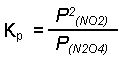• Check to see that the given amounts are measured in appropriate pressure units since Kp is to be .  In this example they are (atmospheres).
• Create an ICE chart and calculate the changes in pressure and equilibrium pressures for each species.
 N2O4 NO2 Initial Pressure (atm)(kpa) 3.00 2.00 Change in Pressure (atm)(kpa) + 0.476 – 0.952 Equilibrium Pressure (atm)(kpa) 3.476 1.048
• Substitute the equilibrium pressures into the expression for Kp and solve for Kp.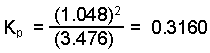This site uses Akismet to reduce spam. Learn how your comment data is processed.